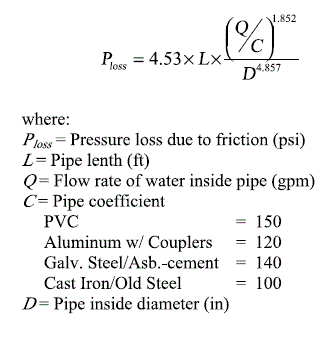You have no items in your shopping cart.
Search

Pipeline Pressure/Friction Loss

The form calculates the pressure or friction loss along a given length of pipeline with a specified inside diameter. Additional pressure losses can occur due to fittings, which add an extra length to the total pipeline. The pressure losses can be estimated using the fitting pressure loss calculator, which gives an equivalent pipe length to add to the overall pipeline length. Learn more about the units used on this page.

Pressure/Friction Loss

Flow Rate In The Pipe:
Pipe Inside Diameter:
Pipe Length:
Pipe Material:
Pressure Loss:

The Equation

This claculator uses this formula to determine the pressure loss due to pipe friction.Reference: Washington State University